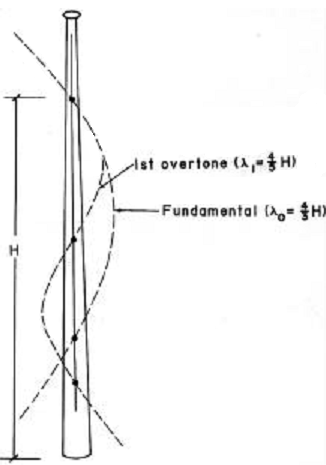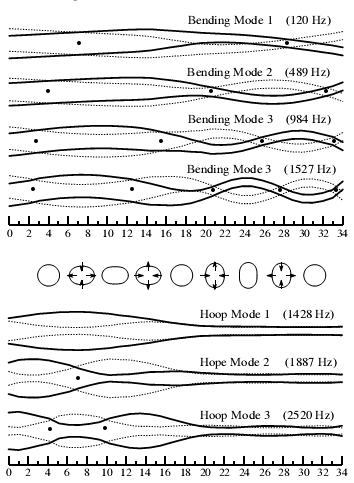Search IntMath
Close

450+ Math Lessons written by Math Professors and Teachers

5 Million+ Students Helped Each Year

1200+ Articles Written by Math Educators and Enthusiasts

Simplifying and Teaching Math for Over 23 Years

# Baseball bat math

By Murray Bourne, 04 Aug 2007

There is some interesting mathematics behind baseball bats.

In a paper on baseball bat physics by Larry Noble, it says:

During the swing, the maximum linear (COP) and rotational velocity of the bat is approximately 33 m/s and 36 r/s, respectively, for college females and 38 m/s and 44 r/s, respectively, for college males.

Some explanations:

"COP" means "center of percussion".
m/s is meters/second

The COP is important because:

When the ball hits the bat at the center of percussion (COP), there is no reaction impulse (shock) at the axis. The impact axis for bats has been shown to be the point under the first knuckle of the top hand.

The paper goes on to describe differences in resonant frequency characteristics between wooden bats and hollow-wall aluminium (aka "aluminum") bats. There is also an analysis of the overtones set up in the bat during the swing:During impact, the vibration behavior of the bat corresponds to that of a free, non-supported bat [...] irregardless of the firmness of the grip.

One of the problems identified in the paper:

Because the primary goal of the power swing is to maximize bat velocity on impact, it is somewhat surprising that maximum bat velocity has repeatedly been found at from .01 to .05 s prior to impact

The paper's conclusion:

The most important mechanical properties of the bat are: (1) mass, (2) moment of inertia, (3) coefficient of restitution (COR), (4) vibrational properties, (5) center of percussion location (COP), and (6) maximum power point location.

The paper involves other applications of mathematics, including:

• Calculus
• Statistics
• Trigonometry
• Functions
• Geometry

## Hoop Frequency

In another paper, Hoop Frequency as a predictor of performance for softball bats, by D. Russell at Kettering University (which is no longer available) we learn that:

Hollow metal and composite baseball and softball bats exhibit two types of vibrational modes, bending modes and hoop modes. The hoop modes are unique to hollow bats and involve only a radial vibration of the barrel of the bat. The lowest frequency hoop mode is responsible for both the "ping" sound of a metal bat and the so-called "trampoline effect".Diagram shows bending mode and hoop mode of a baseball bat. Dots represent nodes.

There are many interesting applications of mathematics in this paper as well:

• Differential equations
• Statistics
• Trigonometry

Baseball bats are an interesting mathematical study. We should start math lessons with such applications and draw the theory from it, rather than trying to rush through applications at the end of a lesson.

See the 1 Comment below.

### One Comment on “Baseball bat math”

1. David says:

Larry Noble is right.velocity of the bat is approximately 33 m/s and 36 r/s, respectively, for college females and 38 m/s and 44 r/s.

### Comment Preview

HTML: You can use simple tags like <b>, <a href="...">, etc.

To enter math, you can can either:

1. Use simple calculator-like input in the following format (surround your math in backticks, or qq on tablet or phone):
a^2 = sqrt(b^2 + c^2)
(See more on ASCIIMath syntax); or
2. Use simple LaTeX in the following format. Surround your math with $$ and $$.
$$\int g dx = \sqrt{\frac{a}{b}}$$
(This is standard simple LaTeX.)

NOTE: You can mix both types of math entry in your comment.